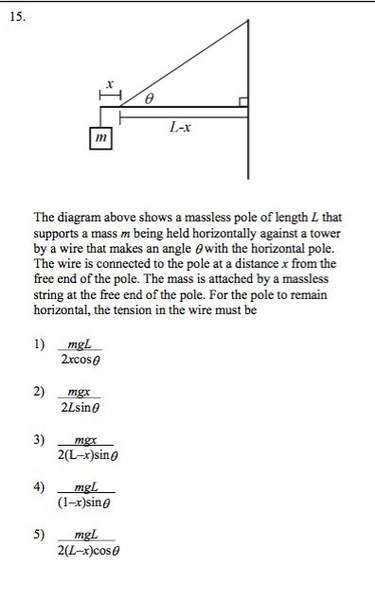# Question on elementary statics multiple choice

• RoboNerd

## Homework Statement## Homework Equations

sum of torques = 0

## The Attempt at a Solution

So I tried to put the torques into the equation:

Sum torques = mgL - T *sin(theta) * (L-x) = 0.

Solving for the T gave me [mgL]/[ (L-x) * sin(theta) ]. This is not an option in the answers.

•